# Mathematics TextbooksCollection Resources(113)

View
Selected filters:
• Community College / Lower Division
• College / Upper Division
• Textbook
• Mathematics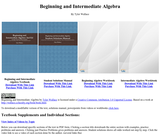Conditions of Use:
No Strings Attached
Rating

Beginning and Intermediate Algebra by Tyler Wallace is a textbook licensed under ...

Beginning and Intermediate Algebra by Tyler Wallace is a textbook licensed under a Creative Commons Attribution 3.0 Unported License. There is also a student guide and supplemental videos for each section.

Subject:
Algebra
Material Type:
Textbook
Provider:
Big Bend Community College
Author:
Tyler Wallace
Date created
28-ربيع الأول-1435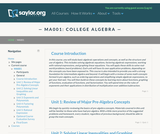Conditions of Use:
Rating

This course is also intended to provide the student with a strong ...

This course is also intended to provide the student with a strong foundation for intermediate algebra and beyond. Upon successful completion of this course, you will be able to: simplify and solve linear equations and expressions including problems with absolute values and applications; solve linear inequalities; find equations of lines; and solve application problems; add, subtract, multiply, and divide various types of polynomials; factor polynomials, and simplify square roots; evaluate, simplify, multiply, divide, add, and subtract rational expressions, and solve basic applications of rational expressions. This free course may be completed online at any time. It has been developed through a partnership with the Washington State Board for Community and Technical Colleges; the Saylor Foundation has modified some WSBCTC materials. (Mathematics 001)

Subject:
Algebra
Material Type:
Assessment
Full Course
Homework/Assignment
Lecture
Syllabus
Textbook
Provider:
The Saylor Foundation
Date created
25-جمادي الأولى-1433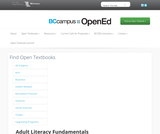Conditions of Use:
No Strings Attached
Rating

: Fundamental mathematics for adult learners. Book 1 includes a Table of ...

: Fundamental mathematics for adult learners. Book 1 includes a Table of Contents, Glossary, Grades Records, Self Tests, Practice Tests and Unit Tests. Ancillary Resources include the Instructor's Manual. This is 1 of a series of 6 books in the ABE Math collection.

Subject:
Education
Mathematics
Material Type:
Textbook
Provider:
BCcampus
Provider Set:
BCcampus Open Textbooks
Author:
Liz Gerard
Wendy Tagami
Date created
7-جمادي الآخرة-1436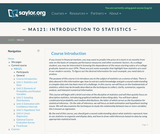Conditions of Use:
Rating

This course covers descriptive statistics, the foundation of statistics, probability and random ...

This course covers descriptive statistics, the foundation of statistics, probability and random distributions, and the relationships between various characteristics of data. Upon successful completion of the course, the student will be able to: Define the meaning of descriptive statistics and statistical inference; Distinguish between a population and a sample; Explain the purpose of measures of location, variability, and skewness; Calculate probabilities; Explain the difference between how probabilities are computed for discrete and continuous random variables; Recognize and understand discrete probability distribution functions, in general; Identify confidence intervals for means and proportions; Explain how the central limit theorem applies in inference; Calculate and interpret confidence intervals for one population average and one population proportion; Differentiate between Type I and Type II errors; Conduct and interpret hypothesis tests; Compute regression equations for data; Use regression equations to make predictions; Conduct and interpret ANOVA (Analysis of Variance). (Mathematics 121; See also: Biology 104, Computer Science 106, Economics 104, Psychology 201)

Subject:
Statistics and Probability
Material Type:
Assessment
Full Course
Homework/Assignment
Lecture
Syllabus
Textbook
Provider:
The Saylor Foundation
Date created
15-ذو الحجة-1432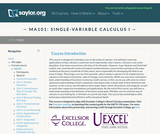Conditions of Use:
Rating

This course is designed to introduce the student to the study of ...

This course is designed to introduce the student to the study of Calculus through concrete applications. Upon successful completion of this course, students will be able to: Define and identify functions; Define and identify the domain, range, and graph of a function; Define and identify one-to-one, onto, and linear functions; Analyze and graph transformations of functions, such as shifts and dilations, and compositions of functions; Characterize, compute, and graph inverse functions; Graph and describe exponential and logarithmic functions; Define and calculate limits and one-sided limits; Identify vertical asymptotes; Define continuity and determine whether a function is continuous; State and apply the Intermediate Value Theorem; State the Squeeze Theorem and use it to calculate limits; Calculate limits at infinity and identify horizontal asymptotes; Calculate limits of rational and radical functions; State the epsilon-delta definition of a limit and use it in simple situations to show a limit exists; Draw a diagram to explain the tangent-line problem; State several different versions of the limit definition of the derivative, and use multiple notations for the derivative; Understand the derivative as a rate of change, and give some examples of its application, such as velocity; Calculate simple derivatives using the limit definition; Use the power, product, quotient, and chain rules to calculate derivatives; Use implicit differentiation to find derivatives; Find derivatives of inverse functions; Find derivatives of trigonometric, exponential, logarithmic, and inverse trigonometric functions; Solve problems involving rectilinear motion using derivatives; Solve problems involving related rates; Define local and absolute extrema; Use critical points to find local extrema; Use the first and second derivative tests to find intervals of increase and decrease and to find information about concavity and inflection points; Sketch functions using information from the first and second derivative tests; Use the first and second derivative tests to solve optimization (maximum/minimum value) problems; State and apply Rolle's Theorem and the Mean Value Theorem; Explain the meaning of linear approximations and differentials with a sketch; Use linear approximation to solve problems in applications; State and apply L'Hopital's Rule for indeterminate forms; Explain Newton's method using an illustration; Execute several steps of Newton's method and use it to approximate solutions to a root-finding problem; Define antiderivatives and the indefinite integral; State the properties of the indefinite integral; Relate the definite integral to the initial value problem and the area problem; Set up and calculate a Riemann sum; Estimate the area under a curve numerically using the Midpoint Rule; State the Fundamental Theorem of Calculus and use it to calculate definite integrals; State and apply basic properties of the definite integral; Use substitution to compute definite integrals. (Mathematics 101; See also: Biology 103, Chemistry 003, Computer Science 103, Economics 103, Mechanical Engineering 001)

Subject:
Calculus
Material Type:
Assessment
Full Course
Homework/Assignment
Syllabus
Textbook
Provider:
The Saylor Foundation
Date created
15-ذو الحجة-1432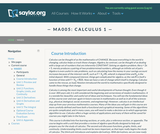Conditions of Use:
Rating

This course begins with a review of algebra specifically designed to help ...

This course begins with a review of algebra specifically designed to help and prepare the student for the study of calculus, and continues with discussion of functions, graphs, limits, continuity, and derivatives. The appendix provides a large collection of reference facts, geometry, and trigonometry that will assist in solving calculus problems long after the course is over. Upon successful completion of this course, the student will be able to: calculate or estimate limits of functions given by formulas, graphs, or tables by using properties of limits and LĺÎĺ_ĺĚĺ_hopitalĺÎĺ_ĺĚĺ_s Rule; state whether a function given by a graph or formula is continuous or differentiable at a given point or on a given interval and justify the answer; calculate average and instantaneous rates of change in context, and state the meaning and units of the derivative for functions given graphically; calculate derivatives of polynomial, rational, common transcendental functions, and implicitly defined functions; apply the ideas and techniques of derivatives to solve maximum and minimum problems and related rate problems, and calculate slopes and rates for function given as parametric equations; find extreme values of modeling functions given by formulas or graphs; predict, construct, and interpret the shapes of graphs; solve equations using NewtonĺÎĺ_ĺĚĺ_s Method; find linear approximations to functions using differentials; festate in words the meanings of the solutions to applied problems, attaching the appropriate units to an answer; state which parts of a mathematical statement are assumptions, such as hypotheses, and which parts are conclusions. This free course may be completed online at any time. It has been developed through a partnership with the Washington State Board for Community and Technical Colleges; the Saylor Foundation has modified some WSBCTC materials. (Mathematics 005)

Subject:
Calculus
Functions
Material Type:
Assessment
Full Course
Syllabus
Textbook
Provider:
The Saylor Foundation
Date created
25-جمادي الأولى-1433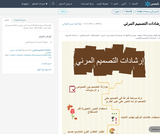Conditions of Use:
Remix and Share
Rating

انفوجرافيك يوضح أهم الأساسيات التي ينبغي مراعاتها لتصميم أي منتج مرئي ( ...

انفوجرافيك يوضح أهم الأساسيات التي ينبغي مراعاتها لتصميم أي منتج مرئي ( انفوجرافيك، عرض، فيديو )

Subject:
Applied Science
Arabic Language and Arts
Arts and Humanities
Career and Technical Education
Education
English Language Arts
History
Law
Life Science
Mathematics
Physical Science
Religion and Islam
Social Science
Material Type:
Activity/Lab
Assessment
Diagram/Illustration
Homework/Assignment
Interactive
Lecture
Lecture Notes
Lesson
Lesson Plan
Module
Teaching/Learning Strategy
Textbook
Provider:
Author:
مها أحمد حسين الزهراني
Date created
22-ربيع الأول-1440Conditions of Use:
No Strings Attached
Rating

Elementary Linear Algebra was written and submitted to the Open Textbook Challenge ...

Elementary Linear Algebra was written and submitted to the Open Textbook Challenge by Dr. Kenneth Kuttler of Brigham Young University. Dr. Kuttler wrote this textbook for use by his students at BYU. According to the introduction of Elementary Linear Algebra, ĺÎĺ_ĺĚĄ_this is intended to be a first course in linear algebra for students who are sophomores or juniors who have had a course in one variable calculus and a reasonable background in college algebra.ĺÎĺ_ĺĚĺÎĺ A solutions manual for the textbook is included.

Subject:
Algebra
Material Type:
Full Course
Textbook
Provider:
The Saylor Foundation
Author:
Kenneth Kuttler
Date created
12-ربيع الثاني-1433Conditions of Use:
Rating

فيديو يوضح أنواع القطوع المخروطية ويشرح القطع المكافئ بشكل مفصل

Subject:
Applied Science
Mathematics
Material Type:
Textbook
Provider:
رياضياتي
Author:
أ.ابراهيم الساحلي
Date created
27-ربيع الأول-1439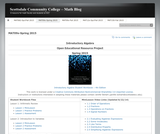Conditions of Use:
Rating

This workbook was created by mathematics instructors at Scottsdale Community College in ...

This workbook was created by mathematics instructors at Scottsdale Community College in Scottsdale, Arizona. It is designed to lead students through Introductory Algebra, and to help them develop a deep understanding of the concepts. The included curriculum is broken into twelve lessons. 303 pages. Also contains links to video mini-lessons.

Subject:
Mathematics
Algebra
Material Type:
Lecture
Textbook
Provider:
Scottsdale Community College
Provider Set:
Scottsdale Community College Open Textbooks
Author:
Donna Guhse
Jenifer Bohart
Judy Sutor
William Meacham
Date created
14-جمادي الآخرة-1436Conditions of Use:
No Strings Attached
Rating

: Fundamental mathematics for adult learners. Book 5 includes a Table of ...

: Fundamental mathematics for adult learners. Book 5 includes a Table of Contents, Glossary, Grades Records, Self Tests, Practice Tests and Unit Tests. Ancillary Resources include the Instructor's Manual. This is 1 of a series of 6 books in the ABE Math collection.

Subject:
Education
Mathematics
Material Type:
Textbook
Provider:
BCcampus
Provider Set:
BCcampus Open Textbooks
Author:
Liz Gerard
Wendy Tagami
Date created
7-جمادي الآخرة-1436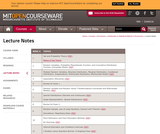Rating

This course is a self-contained introduction to statistics with economic applications. Elements ...

This course is a self-contained introduction to statistics with economic applications. Elements of probability theory, sampling theory, statistical estimation, regression analysis, and hypothesis testing. It uses elementary econometrics and other applications of statistical tools to economic data. It also provides a solid foundation in probability and statistics for economists and other social scientists.

Subject:
Mathematics
Economics
Material Type:
Full Course
Textbook
Provider:
M.I.T.
Provider Set:
M.I.T. OpenCourseWare
Author:
MIT
Prof. Sara Ellison
Date created
14-محرم-1436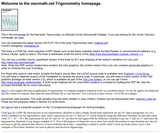Conditions of Use:
Remix and Share
Rating

Book description: This is a text on elementary trigonometry, designed for students ...

Book description: This is a text on elementary trigonometry, designed for students who have completed courses in high-school algebra and geometry. Though designed for college students, it could also be used in high schools. The traditional topics are covered, but a more geometrical approach is taken than usual. Also, some numerical methods (e.g. the secant method for solving trigonometric equations) are discussed. A brief tutorial on using Gnuplot to graph trigonometric functions is included.

There are 495 exercises in the book, with answers and hints to selected exercises.

Subject:
Trigonometry
Material Type:
Textbook
Provider:
Schoolcraft College
Author:
Michael Corral
Date created
21-ربيع الأول-1434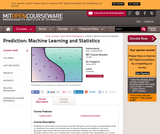Conditions of Use:
Remix and Share
Rating

Prediction is at the heart of almost every scientific discipline, and the ...

Prediction is at the heart of almost every scientific discipline, and the study of generalization (that is, prediction) from data is the central topic of machine learning and statistics, and more generally, data mining. Machine learning and statistical methods are used throughout the scientific world for their use in handling the "information overload" that characterizes our current digital age. Machine learning developed from the artificial intelligence community, mainly within the last 30 years, at the same time that statistics has made major advances due to the availability of modern computing. However, parts of these two fields aim at the same goal, that is, of prediction from data. This course provides a selection of the most important topics from both of these subjects.

Subject:
Statistics and Probability
Material Type:
Full Course
Textbook
Provider:
M.I.T.
Provider Set:
M.I.T. OpenCourseWare
Author:
Cynthia Rudin
Date created
3-ذو القعدة-1434Conditions of Use:
No Strings Attached
Rating

: Fundamental mathematics for adult learners. Book 4 includes a Table of ...

: Fundamental mathematics for adult learners. Book 4 includes a Table of Contents, Glossary, Grades Records, Self Tests, Practice Tests and Unit Tests. Ancillary Resources include the Instructor's Manual. This is 1 of a series of 6 books in the ABE Math collection.

Subject:
Education
Mathematics
Material Type:
Textbook
Provider:
BCcampus
Provider Set:
BCcampus Open Textbooks
Author:
Liz Gerard
Wendy Tagami
Date created
7-جمادي الآخرة-1436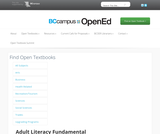Conditions of Use:
No Strings Attached
Rating

: Fundamental mathematics for adult learners. Book 2 includes a Table of ...

: Fundamental mathematics for adult learners. Book 2 includes a Table of Contents, Glossary, Grades Records, Self Tests, Practice Tests and Unit Tests. Ancillary Resources include the Instructor's Manual. This is 1 of a series of 6 books in the ABE Math collection.

Subject:
Education
Mathematics
Material Type:
Textbook
Provider:
BCcampus
Provider Set:
BCcampus Open Textbooks
Author:
Liz Gerard
Wendy Tagami
Date created
7-جمادي الآخرة-1436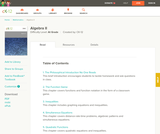Conditions of Use:
Remix and Share
Rating

Algebra II provides an introduction to Advanced Algebra topics.

Subject:
Algebra
Material Type:
Textbook
Provider:
CK-12 Foundation
Provider Set:
CK-12 FlexBook
Date created
11-شعبان-1434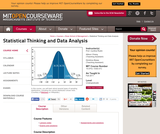Conditions of Use:
Remix and Share
Rating

This course is an introduction to statistical data analysis. Topics are chosen ...

This course is an introduction to statistical data analysis. Topics are chosen from applied probability, sampling, estimation, hypothesis testing, linear regression, analysis of variance, categorical data analysis, and nonparametric statistics.

Subject:
Statistics and Probability
Material Type:
Full Course
Textbook
Provider:
M.I.T.
Provider Set:
M.I.T. OpenCourseWare
Author:
Allison Chang
Cynthia Rudin
Dimitrios Bisias
Date created
28-شوال-1434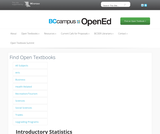Conditions of Use:
No Strings Attached
Rating

This book is meant to be a textbook for a standard one-semester ...

This book is meant to be a textbook for a standard one-semester introductory statistics course for general education students.Over time the core content of this course has developed into a well-defined body of material that is substantial for a one-semester course. The authors believe that the students in this course are best served by a focus on the core material and not by an exposure to a plethora of peripheral topics. Therefore in writing this book we have sought to present material that comprises fully a central body of knowledge that is defined according to convention, realistic expectation with respect to course duration and students maturity level, and our professional judgment and experience.

Subject:
Statistics and Probability
Material Type:
Textbook
Provider:
BCcampus
Provider Set:
BCcampus Faculty Reviewed Open Textbooks
Author:
Douglas S. Shafer
Zhiyi Zhang
Date created
5-جمادي الآخرة-1436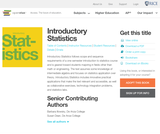Conditions of Use:
No Strings Attached
Rating

Introductory Statistics follows scope and sequence requirements of a one-semester introduction to ...

Introductory Statistics follows scope and sequence requirements of a one-semester introduction to statistics course and is geared toward students majoring in fields other than math or engineering. The text assumes some knowledge of intermediate algebra and focuses on statistics application over theory. Introductory Statistics includes innovative practical applications that make the text relevant and accessible, as well as collaborative exercises, technology integration problems, and statistics labs.

Subject:
Statistics and Probability
Material Type:
Textbook
Provider:
Rice University
Provider Set:
OpenStax College
Author:
Barbara Ilowsky
Susan Dean
Date created
4-شوال-1437